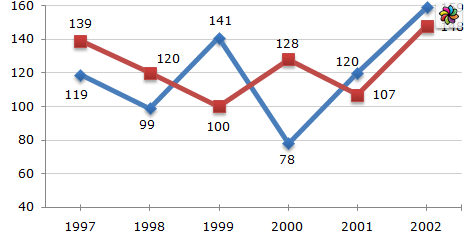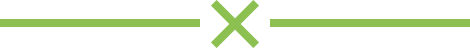# Unit – 4 : graphs and charts for business data

##### Unit – 4 :Graphs and Charts For Business Data
• Graph is a pictorial representation of data,which has at least two dimensional relationship.It has two axes X and Y.It is used to represent variation in values over a period of time.
• Charts are used to give information about the frequency of different quantities in a single pictorial representation.
##### Types of Charts
• Column Chart : Column chart shows a bar chart or bar graph with vertical bars.The height of each bar is proportional to its value. Categories are displayed horizontally( X axis) and Values are vertically(Y axis).

• Bar Charts:   Barc Charts shows horizontal bars. The length of each bar is proportional to its value.

• Line Chart: The values of each data series in a line chart can be connected by a line. X axis shows the categories and Y axis shows values as points.It is used to display trends over a period of time.
•• Pie Chart:  A Pie chart displays the contribution of each value to a total. It represents multiple sub-group of a single variable.

• Exploded Pie Chart : In this type of chart one or several slices are separated from other.

• Donut Chart /Doughnut Chart: It is a Pie chart with a hole in the middle and it can display more than one series of data.

•   Area Chart: Area chart shows values as points on the Y axis and categories on X axis. The values of each data series are connected by a line,the area between two lines is filled with a colour.

• Scatter Chart( XY Chart): In this chart both axis display values.It is used to show the relationship among two variables.

• Radar Charat : It also known as net chart or Star chart.A Net Chart has separate  axis for each data category, and the axes extended from the centre of the chart.

##### Elements of a Chart /Graph

The different parts that make up a chart re referred to as elements.

• Chart Area: Entire area of the chart, which includes labels, data, axis etc.
• Chart Wall : It is the area in which chart is plotted.
• Chart Main Title/Subtitle: This is the explanatory heading at the top of the chart.
• Axes : A line that serves as a major reference for plotting data. X-axis, Y-axis and Z-axis.
• Data Point/ Data Marker: Individual values plotted in a chart and represented by bar,Column,line etc.
• Data Series : A group of data points.
• Legend : It is an identifier of a piece of information shown in the chart/graph.
• Data label : The value of the data series plotted in a chart is known a data label.
• Grid lines:These are the vertical and horizontal lines that appear in a chart.
##### Steps for Chart/Graph
1. Enter the data which needed to create the chart/Graph.
2. Select the data is needed for plotting the chart
3. Plotting the chart, Insert ->Chart.
4. Select the chart type, click Next
5. In Data Range , select “Data series in column”, “First row as label” and “First column as label”. And click next.
6. Under chart element enter the “Title” and enter labels for X axis and  Y axis then click finish.